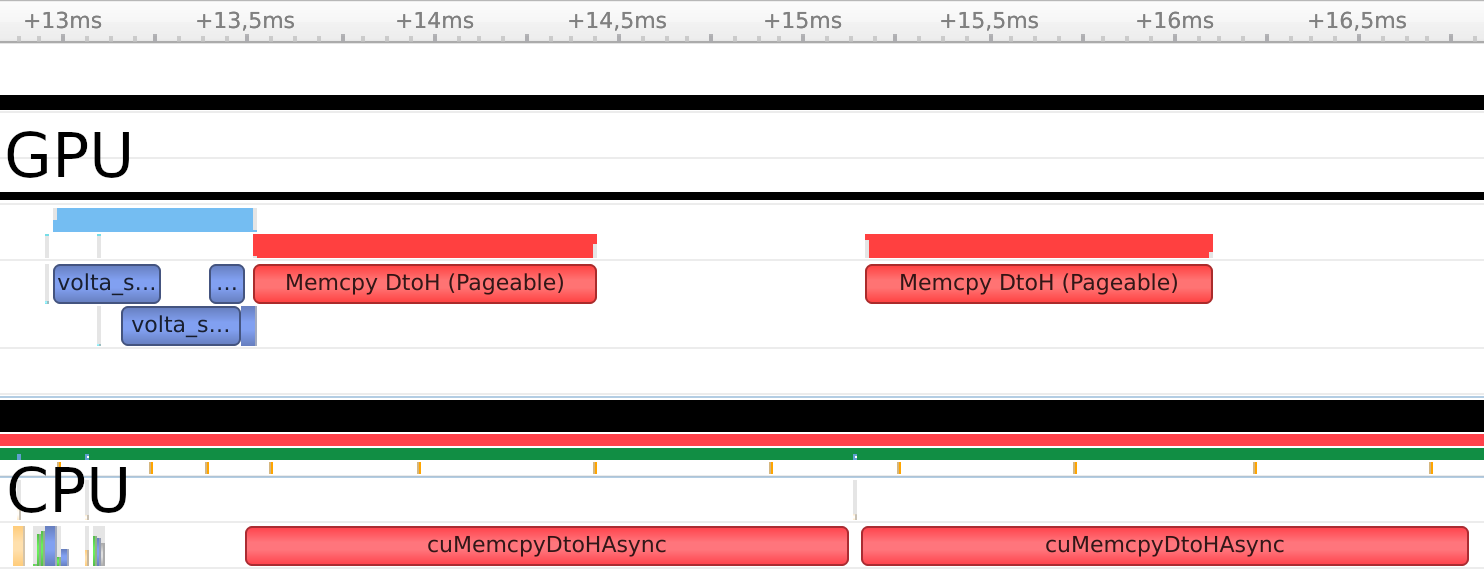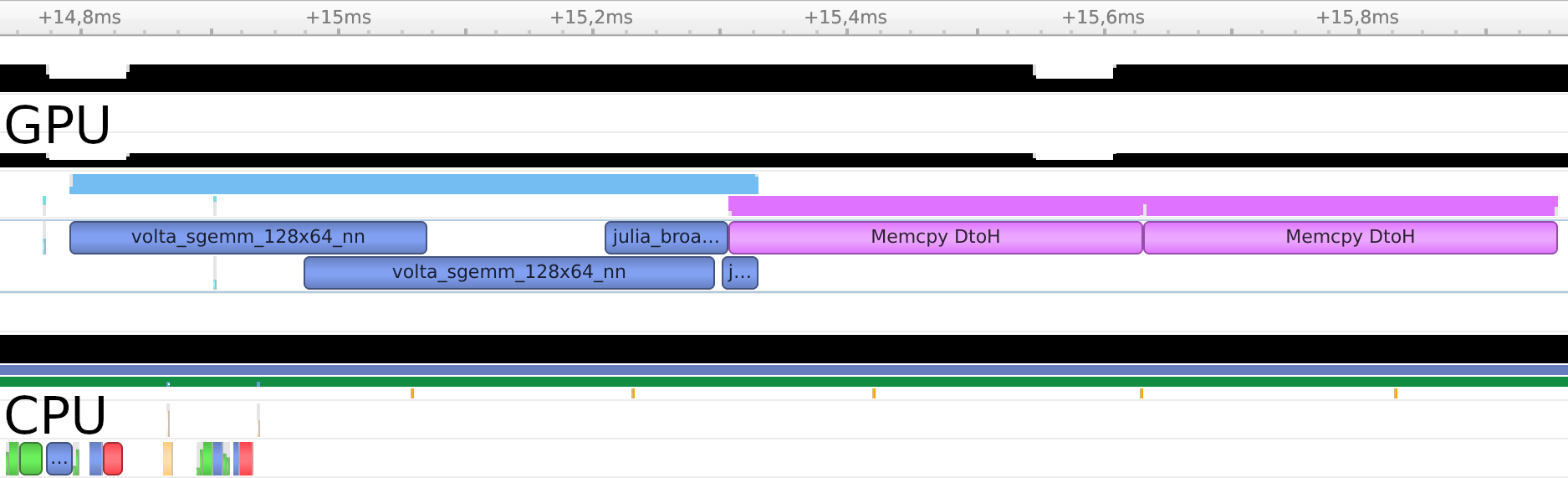CUDA.jl can be used with Julia tasks and threads, offering a convenient way to work with multiple devices, or to perform independent computations that may execute concurrently on the GPU.

Each Julia task gets its own local CUDA execution environment, with its own stream, library handles, and active device selection. That makes it easy to use one task per device, or to use tasks for independent operations that can be overlapped. At the same time, it's important to take care when sharing data between tasks.

For example, let's take some dummy expensive computation and execute it from two tasks:

# an expensive computation
function compute(a, b)
c = a * b             # library call
broadcast!(sin, c, c) # Julia kernel
c
end

function run(a, b)
results = Vector{Any}(undef, 2)

# computation
@sync begin
@async begin
results = Array(compute(a,b))
nothing # JuliaLang/julia#40626
end
@async begin
results = Array(compute(a,b))
nothing # JuliaLang/julia#40626
end
end

# comparison
results == results
end

We use familiar Julia constructs to create two tasks and re-synchronize afterwards (@async and @sync), while the dummy compute function demonstrates both the use of a library (matrix multiplication uses CUBLAS) and a native Julia kernel. The function is passed three GPU arrays filled with random numbers:

function main(N=1024)
a = CUDA.rand(N,N)
b = CUDA.rand(N,N)

# make sure this data can be used by other tasks!
synchronize()

run(a, b)
end

The main function illustrates how we need to take care when sharing data between tasks: GPU operations typically execute asynchronously, queued on an execution stream, so if we switch tasks and thus switch execution streams we need to synchronize() to ensure the data is actually available.

Using Nsight Systems, we can visualize the execution of this example:You can see how the two invocations of compute resulted in overlapping execution. The memory copies, however, were executed in serial. This is expected: Regular CPU arrays cannot be used for asynchronous operations, because their memory is not page-locked. For most applications, this does not matter as the time to compute will typically be much larger than the time to copy memory.

If your application needs to perform many copies between the CPU and GPU, it might be beneficial to "pin" the CPU memory so that asynchronous memory copies are possible. This operation is expensive though, and should only be used if you can pre-allocate and re-use your CPU buffers. Applied to the previous example:

function run(a, b)
results = Vector{Any}(undef, 2)

# pre-allocate and pin destination CPU memory
results = Mem.pin(Array{eltype(a)}(undef, size(a)))
results = Mem.pin(Array{eltype(a)}(undef, size(a)))

# computation
@sync begin
@async begin
copyto!(results, compute(a,b))
nothing # JuliaLang/julia#40626
end
@async begin
copyto!(results, compute(a,b))
nothing # JuliaLang/julia#40626
end
end

# comparison
results == results
endThe profile reveals that the memory copies themselves could not be overlapped, but the first copy was executed while the GPU was still active with the second round of computations. Furthermore, the copies executed much quicker – if the memory were unpinned, it would first have to be staged to a pinned CPU buffer anyway.

Use of tasks can be easily extended to multiple threads with functionality from the Threads standard library:

function run(a, b)
results = Vector{Any}(undef, 2)

# computation
@sync begin
results = Array(compute(a,b))
nothing # JuliaLang/julia#40626
end
results = Array(compute(a,b))
nothing # JuliaLang/julia#40626
end
end

# comparison
results == results
end

By using the Threads.@spawn macro, the tasks will be scheduled to be run on different CPU threads. This can be useful when you are calling a lot of operations that "block" in CUDA, e.g., memory copies to or from unpinned memory. Generally though, operations that synchronize GPU execution (including the call to synchronize itself) are implemented in a way that they yield back to the Julia scheduler, to enable concurrent execution without requiring the use of different CPU threads.

Warning

Use of multiple threads with CUDA.jl is a recent addition, and there may still be bugs or performance issues.